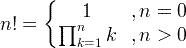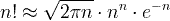# Factorial

## What is factorial (n!)

When you saw an exclamation point in maths for the first time, you probably got shocked or even thought that there was some kind of mistake or typo. But the reality is different: this exclamation point in maths is called the factorial or n-factorial. The factorial is a reasonably unknown operator that can, in fact, be viewed more as an abbreviation than an actual operator, at least at the beginning.

It is important not to confuse the factorial with the prime factorization of a number, which is a way of obtaining the prime numbers that, when multiplied together, give your number. Prime factorization has its uses in maths and is arguably more well known than the n-factorial. Part of the reason for the popularity of prime factorization is its usefulness when calculating Greatest Common Factor (GCM) and the Least Common Multiplier (LCM), but we digress.

To understand what the factorial does or means, we should start with an example. We could choose any number n and calculate its n-factorial value, but it's best to choose a relatively small number, so let's use 5-factorial.

5! = 5 * 4 * 3 * 2 * 1 = 120

From this example, you can see that it is not rocket science, and you might even take a guess at the factorial formula. You can also understand why this exclamation point in maths can be regarded as an abbreviation since it is not a new operation but rather a collection of multiplications. In short, and somewhat informally, we can define the factorial as the multiplication of all the positive integers smaller than and equal to the given number.

Playing a bit with this, we can see that for 5-factorial, we can relate it to the 4-factorial in a straightforward way:

5! = 5 * (4 * 3 * 2 * 1) = 5 * 4!

This kind of relationship between n-factorials with a different n is the basis of the mathematical formula that defines the factorial operation, as we will see in the next section.

The factorial operation is not used everywhere in maths, but it is essential in statistics and probability problems. In those cases, especially when one has to deal with permutations or combinatorics, the n-factorial appears almost all the time. In the following sections of our factorial calculator, we will see real-world examples of problems that require the usage of factorials and the factorial formula.

So the factorial of n is denoted by n! and calculated by the product of integer numbers from 1 to n.

For n>0,

n! = 1×2×3×4×...×n

For n=0,

0! = 1

### Factorial definition formula#### Examples:

1! = 1

2! = 1×2 = 2

3! = 1×2×3 = 6

4! = 1×2×3×4 = 24

5! = 1×2×3×4×5 = 120

### Recursive factorial formula

n! = n×(n-1)!

#### Example:

5! = 5×(5-1)! = 5×4! = 5×24 = 120

### Stirling's approximation#### Example:

5! ≈ √2π5⋅55⋅e-5 = 118.019

Number
n
Factorial
n!
0 1
1 1
2 2
3 6
4 24
5 120
6 720
7 5040
8 40320
9 362880
10 3628800
11 3.991680x107
12 4.790016x108
13 6.227021x109
14 8.717829x1010
15 1.307674x1012
16 2.092279x1013
17 3.556874x1014
18 6.402374x1015
19 1.216451x1017
20 2.432902x1018

## C program for factorial calculation

double factorial(unsigned int n) {

double fact=1.0;

if( n > 1 )

for(unsigned int k=2; k<=n; k++)

fact = fact*k;

return fact;

## Explanation of zero factorial

We can understand why many of us have a hard time accepting the fact that the value of zero factorial is equal to one. It comes across as an absurd statement that there’s no way it can be true. We have a common perception of zero for being notorious because there’s something about it that can make any number associated with it either vanish or misbehave.

For instance, a large number such as 1,000 multiplied by zero becomes zero. It disappears! On the other hand, a nice number such as 5 divided by zero becomes undefined. It misbehaves. So it is okay to be skeptical why zero “suddenly” becomes one, a nice number, after treating it with some special operation.

There are other ways to show why the statement is true. For this one, we will use the definition of factorial itself. To be honest, with this method the justification is simple and requires little math.

Let $n$ be a whole number, where $n!$ is defined as the product of all whole numbers less than $n$ and including $n$ itself.

What it means is that you first start writing the whole number $n$ then count down until you reach the whole number $1$.

The general formula of factorial can be written in fully expanded form as

or in partially expanded form as

We know with absolute certainty that 1!=1, where $n=1$. If we substitute that value of $n$ into the second formula which is the partially expanded form of $n!$, we obtain the following:

For the equation to be true, we must force the value of zero factorial to equal 1, and no other. Otherwise, 1!≠1 which is a contradiction.

So yes, 0! = 1 is correct because mathematicians agreed to define it that way (nothing more and nothing less) in order to be consistent with the rest of mathematics. (Source: Chilimath.com)

Currently, we have around 5612 calculators, conversion tables and usefull online tools and software features for students, teaching and teachers, designers and simply for everyone.